Question

Which of the following complexes has the largest crystal field splitting of the d orbitals? A. [Fe(H2O)6]3+ B. [FeF6]3– C. [Fe(NH3)6]3+ D. [FeI6]3– E. [Fe(CO)6]3+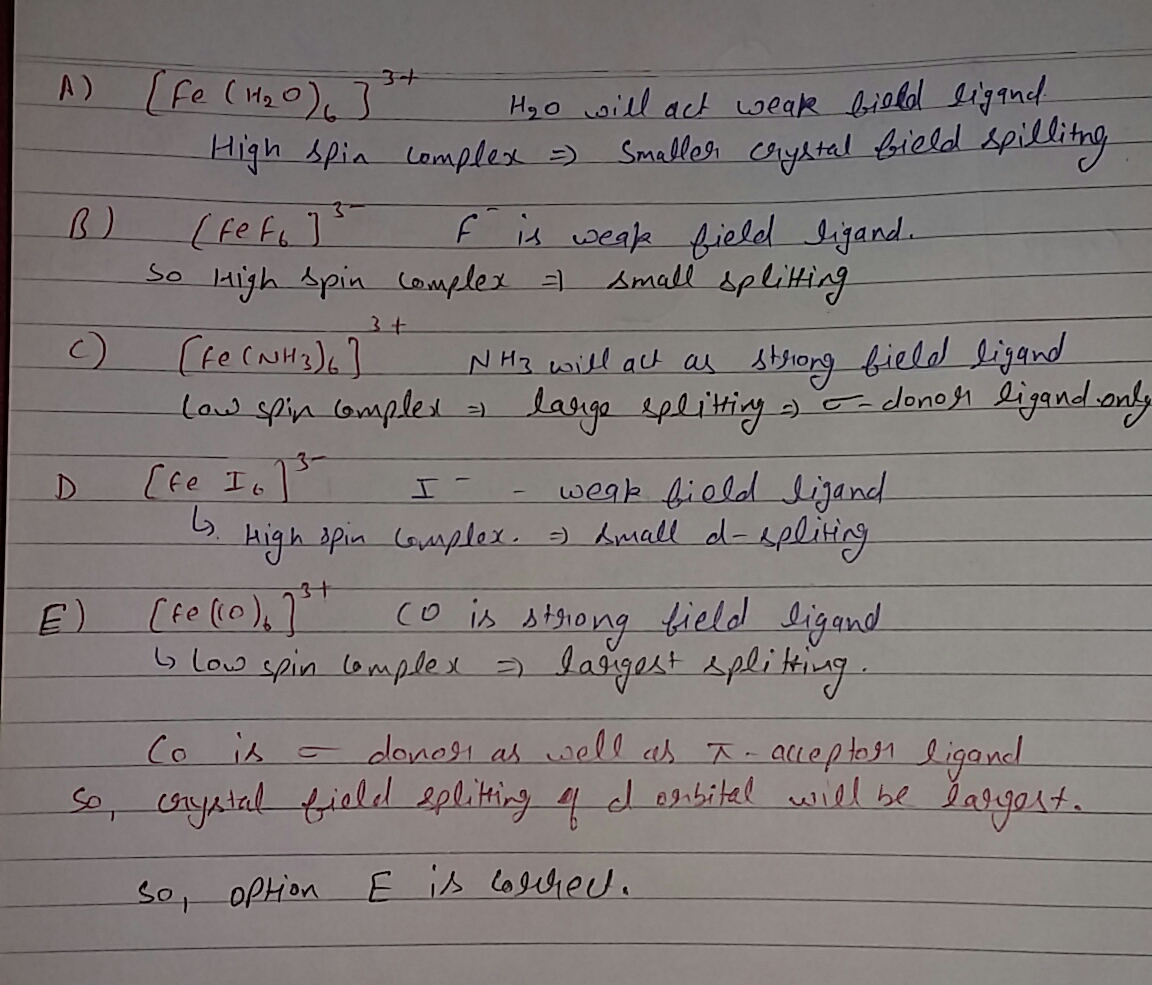#### Earn Coins

Coins can be redeemed for fabulous gifts.

Similar Homework Help Questions
• ### 2. a. Draw and show the crystal field splitting of the d orbitals in an octahedral...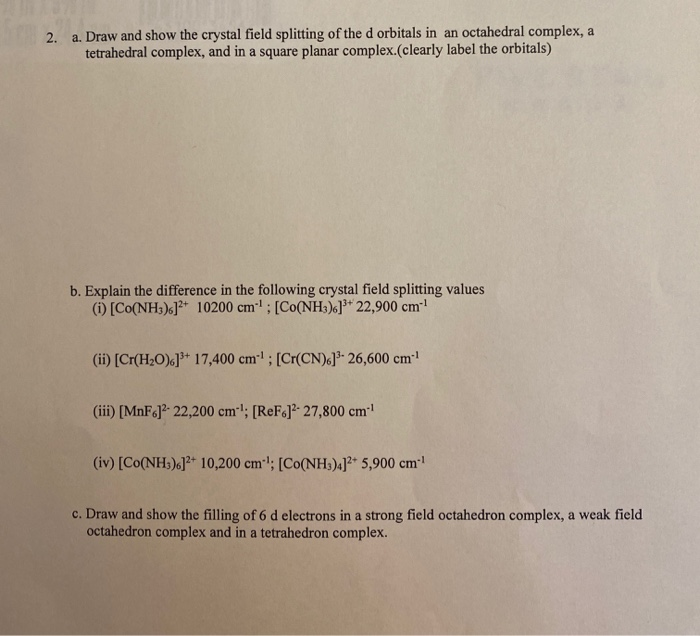2. a. Draw and show the crystal field splitting of the d orbitals in an octahedral complex, a tetrahedral complex, and in a square planar complex.(clearly label the orbitals) b. Explain the difference in the following crystal field splitting values (1) Co(NH3).]2+ 10200 cm"! : [CO(NH3).]* 22,900 cm (ii) [Cr(H20).]** 17,400 cm. ; [Cr(CN).]3-26,600 cm (iii) [MnF6]?- 22,200 cm-"; [ReF.]?- 27,800 cm- (iv) [Co(NH3).]2+ 10,200 cm '; [Co(NH3)4]2+ 5,900 cm c. Draw and show the filling of 6 d electrons...

• ### Which ion would you expect to have the largest crystal field splitting, ?

Which ion would you expect to have the largest crystal field splitting, ?A. [Rh(CN)6]4-B. [Rh(CN)6]3-C. [Rh(H2O)6]2+D. [Rh(H2O)6]3+

• ### Predict the electron configurations of the d-orbitals (crystal field diagrams) for the...

Predict the electron configurations of the d-orbitals (crystal field diagrams) for the transition-metaltetrahedral and square planar complexes of [Fe(H2O)4]2+. Then, do the same for [Fe(CO)4]2+. Explain howspectroscopy could potentially be used to distinguish them.

• ### 16.15. For each pair of complexes, predict which will have the larger CF splitting parameter: a....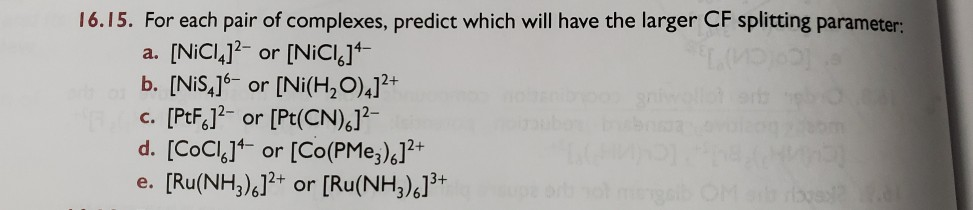16.15. For each pair of complexes, predict which will have the larger CF splitting parameter: a. [NiC14]2- or [NiCic]4- b. [NiS4]6- or [Ni(H2O),]2+ c. [PtF.12- or [Pt(CN),12- d. [CoCl.]- or [Co(PMez).]2+ e. [Ru(NH3).]2+ or [Ru(NH3).]3+

• ### ligand field will cause splitting of energy levels of 3d orbitals of the central metal ion.............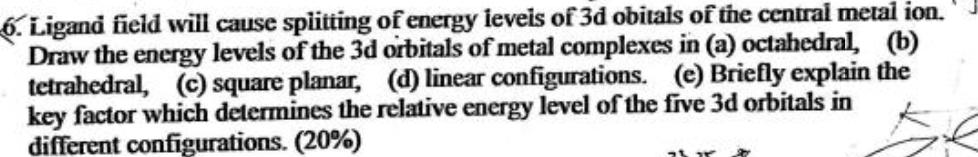ligand field will cause splitting of energy levels of 3d orbitals of the central metal ion.......... splitting of energy ieveis of 3d obitals of the central metal ion. 6. Ligand field will cause Draw the energy levels of the 3d ortbitals of metal complexes in (a) octahedral, (b) tetrahedral, (c) square planar, (d) linear configurations. (e) Briefly explain the key factor which determines the relative energy level of the five 3d orbitals in different configurations. (20 % ) splitting of...

• ### Part A (2 marks) In the following pairs of complexes, which do you expect to have...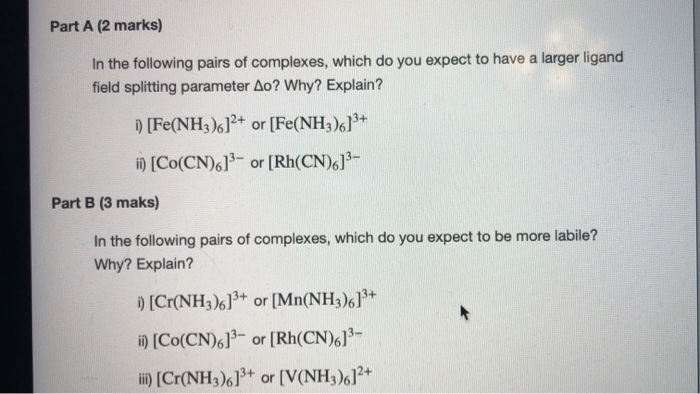Part A (2 marks) In the following pairs of complexes, which do you expect to have a larger ligand field splitting parameter Ao? Why? Explain? i) [Fe(NH3)612+ or [Fe(NH3). 13+ ii) (Co(CN)613- or [Rh(CN)613- Part B (3 maks) In the following pairs of complexes, which do you expect to be more labile? Why? Explain? ) [Cr(NH3)613+ or [Mn(NH3)6]3+ ii) (Co(CN)613- or [Rh(CN)613- ii) [Cr(NH3).]3+ or [V(NH3).]2+

• ### Crystal Field Question. I understand that octahedral shapes have larger splitting energies then in tetrahedral complexes....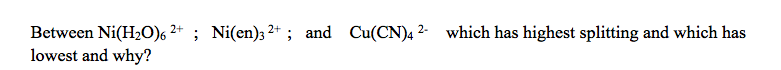Crystal Field Question. I understand that octahedral shapes have larger splitting energies then in tetrahedral complexes. And it would take more energy to split more electrons. So would the order be from Highest to Lowest: B>A>C where A, B, C are the compounds listed in their respective orders. Between Ni(H20)s2+ ; Ni(en)32+; and lowest and why? Cu(CN)42- which has highest splitting and which has

• ### The complex [Fe(H2O)6]3+ has a maximum absorbance at 724 nm. a.Calculate the crystal field splitting energy,...

The complex [Fe(H2O)6]3+ has a maximum absorbance at 724 nm. a.Calculate the crystal field splitting energy, in kJ, for the complex. b.Predict the colour of the complex.

• ### Date: 1117 mework 10 - Ch. 21-23 A) CN B)NO c)r D) CO Name: E ) en 24. Place the ligands, CN-, Br., H2O, and OH-in o...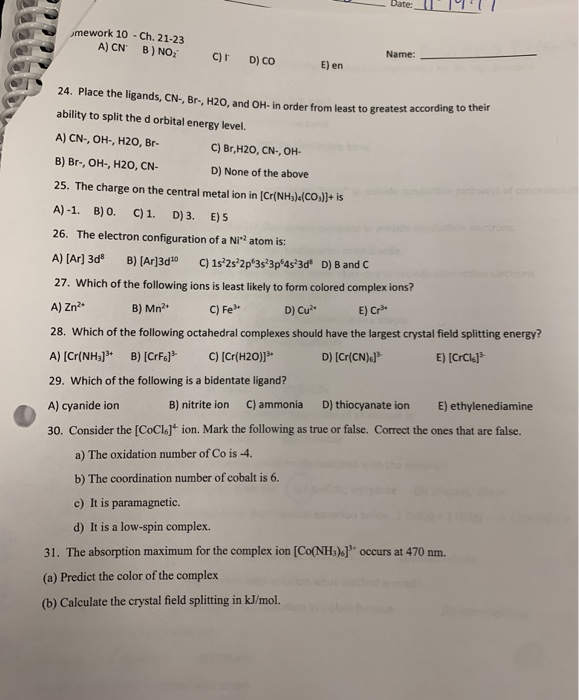Date: 1117 mework 10 - Ch. 21-23 A) CN B)NO c)r D) CO Name: E ) en 24. Place the ligands, CN-, Br., H2O, and OH-in order from least to greatest according ability to split the d orbital energy level. A) CN-, OH, H2O, Br- C) Br,H20, CN-, OH- B) Br., OH, H2O, CN- D) None of the above 25. The charge on the central metal ion in (Cr(NH3)(CO3)2+ is A)-1. B) 0. C) 1. D) 3. E) 5 26....

• ### Question 2 1 pts In tetrahedral crystal field splitting, which orbitals are of equal, but highest...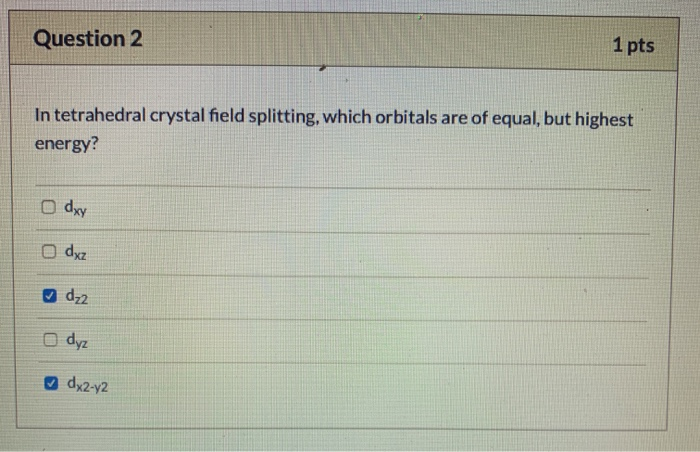Question 2 1 pts In tetrahedral crystal field splitting, which orbitals are of equal, but highest energy? o dxy o dxz dz2 o dyz dx2-y2